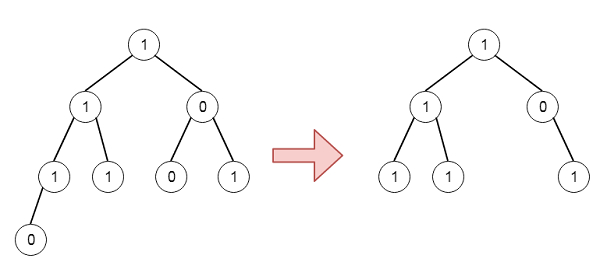# Program to pruning a given binary tree in C++

Suppose we have a binary tree, where every node's value is either a 0 or a 1. We have to find the same tree where every subtree not containing a 1 has been deleted. So if the tree is like −To solve this, we will follow these steps −

• Define a recursive method solve(), this will take the node. the method will be like −

• If node is null, then return null

• left of node := solve(left of node)

• right of node := solve(right of node)

• if left of node is null and right of node is also null and node value is 0, then return null

• return node

Let us see the following implementation to get better understanding −

## Example

Live Demo

#include <bits/stdc++.h>
using namespace std;
class TreeNode{
public:
int val;
TreeNode *left, *right;
TreeNode(int data){
val = data;
left = NULL;
right = NULL;
}
};
void inorder(TreeNode *root){
if(root){
inorder(root->left);
cout << root->val << ", ";
inorder(root->right);
}
}
class Solution {
public:
TreeNode* pruneTree(TreeNode* node) {
if(!node)return NULL;
node->left = pruneTree(node->left);
node->right = pruneTree(node->right);
if(!node->left && !node->right && !node->val){
return NULL;
}
return node;
}
};
main(){
TreeNode *root = new TreeNode(1);
root->left = new TreeNode(1);
root->right = new TreeNode(0);
root->left->left = new TreeNode(1);
root->left->right = new TreeNode(1);
root->right->left = new TreeNode(0);
root->right->right = new TreeNode(1);
root->left->left->left = new TreeNode(0);
Solution ob;
inorder(ob.pruneTree(root));
}

## Input

TreeNode *root = new TreeNode(1);
root−>left = new TreeNode(1);
root−>right = new TreeNode(0);
root−>left−>left = new TreeNode(1);
root−>left−>right = new TreeNode(1);
root−>right−>left = new TreeNode(0);
root−>right−>right = new TreeNode(1);
root−>left−>left−>left = new TreeNode(0);

## Output

1, 1, 1, 1, 0, 1,